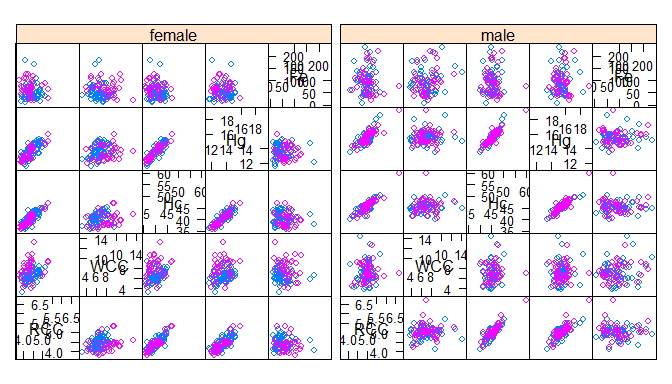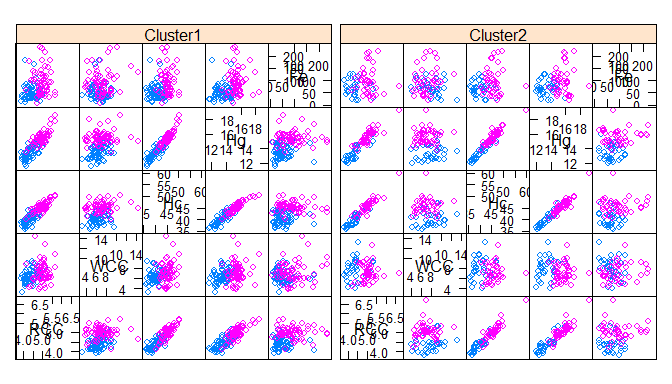# MoEClust: Gaussian Parsimonious Clustering Models with Gating and Expert Network Covariates and a Noise Component

## Introduction

MoEClust is an R package which fits finite Gaussian Mixtures of Experts models using a range of parsimonious covariance parameterisations via the EM/CEM algorithm, ie. allows incorporation of covariates into the mixing proportions and/or Gaussian densities of finite Gaussian mixture models under the various parsimonious covariance parameterisations in the GPCM famly (e.g. mclust). These models were introduced by Murphy and Murphy (2018). The package also facilitates the inclusion of an additional noise component, and allows visualisation of Gaussian mixture of experts models with parsimonious covariance parameterisations using generalised pairs plots.

The most important function in the MoEClust package is: MoE_clust, for fitting the model via the EM/CEM algorithm with gating and/or expert network covariates, supplied via formula interfaces. MoE_compare is provided for conducting model selection between different results from MoE_clust using different covariate combinations &/or initialisation strategies, etc.

MoE_stepwise is provided for conducting a greedy forward stepwise search to identify the optimal model in terms of the number of components, GPCM covariance type, and the subsets of gating/expert network covariates.

MoE_control allows supplying additional arguments to MoE_clust and MoE_stepwise which govern, among other things, controls on the inclusion of an additional noise component and controls on the initialisation of the allocations for the EM/CEM algorithm.

A dedicated plotting function exists for visualising the results using generalised pairs plots, for examining the gating network, and/or log-likelihood, and/or clustering uncertainties, and/or graphing model selection criteria values. The generalised pairs plots (MoE_gpairs) visualise all pairwise relationships between clustered response variables and associated continuous, categorical, and/or ordinal covariates in the gating &/or expert networks, coloured according to the MAP classification, and also give the marginal distributions of each variable (incl. the covariates) along the diagonal.

An as.Mclust method is provided to coerce the output of class "MoEClust" from MoE_clust to the "Mclust" class, to facilitate use of plotting and other functions for the "Mclust" class within the mclust package. As per mclust, MoEClust also facilitates modelling with an additional noise component (with or without the mixing proportion for the noise component depending on covariates). Finally, a predict method is provided for predicting the fitted response and probability of cluster membership (and by extension the MAP classification) for new data, in the form of new covariates and new response data, or new covariates only.

Other functions also exist, e.g. MoE_crit, MoE_dens, MoE_estep, and aitken, which are all used within MoE_clust but are nonetheless made available for standalone use. The package also contains two data sets: ais and CO2data.

If you find bugs or want to suggest new features please visit the MoEClust GitHub issues page. This vignette aims to demonstrate the MoEClust models via application to well-known univariate and multivariate data sets provided with the package.

### Installing MoEClust

MoEClust will run in Windows, Mac OS X or Linux. To install it you first need to install R. Installing Rstudio as a nice desktop environment for using R is also recommended.

Once in R you can type at the R command prompt:

install.packages('devtools')
devtools::install_github('Keefe-Murphy/MoEClust')

to install the latest development version of the package from the MoEClust GitHub page.

To instead install the latest stable official release of the package from CRAN go to R and type:

install.packages('MoEClust')

In either case, if you then type:

library(MoEClust)

it will load in all the MoEClust functions.

The GitHub version contains a few more features but some of these may not yet be fully tested, and occasionally this version might be liable to break when it is in the process of being updated.

## CO2 Data

Load the CO2 data.

data(CO2data)
CO2   <- CO2data$CO2 GNP <- CO2data$GNP

Fit various MoEClust mixture models to cluster the CO2 data, allowing the GNP variable enter the gating &/or expert networks, or neither, via a formula interface. Also consider models with equal mixing proportions. Note that for models with covariates in the gating network, or models with equal mixing proportions, we don’t need to fit single-component models (though it could be done!) as this would merely duplicate the single-component models within m1 and m3, respectively.

m1    <- MoE_clust(CO2, G=1:3, verbose=FALSE)
m2    <- MoE_clust(CO2, G=2:3, gating= ~ GNP, verbose=FALSE)
m3    <- MoE_clust(CO2, G=1:3, expert= ~ GNP, verbose=FALSE)
m4    <- MoE_clust(CO2, G=2:3, gating= ~ GNP, expert= ~ GNP, verbose=FALSE)
m5    <- MoE_clust(CO2, G=2:3, equalPro=TRUE, verbose=FALSE)
m6    <- MoE_clust(CO2, G=2:3, expert= ~ GNP, equalPro=TRUE, verbose=FALSE)

Choose the best model among these.

comp  <- MoE_compare(m1, m2, m3, m4, m5, m6, optimal.only=TRUE)

See if a better model can be found using greedy forward stepwise selection. Conduct a stepwise search on the same data

(mod1 <- MoE_stepwise(CO2, GNP, verbose=FALSE))
## ---------------------------------------------------------------------
## Comparison of Gaussian Parsimonious Clustering Models with Covariates
## Data: CO2
## Ranking Criterion: BIC
## Optimal Only: TRUE
## ---------------------------------------------------------------------
##
##  rank MoENames modelNames G df iters      bic      icl      aic  loglik gating expert algo equalPro
##     1   Step_4          E 3  7    18   -155.2 -159.061 -145.875 -65.937   None   ~GNP   EM     TRUE
##     2   Step_3          V 2  7    11 -157.205 -160.036  -147.88  -66.94   None   ~GNP   EM    FALSE
##     3   Step_2          E 2  4    19 -163.164 -163.911 -157.835 -74.917   None   None   EM    FALSE
##     4   Step_1          E 1  2     1 -163.905 -163.905  -161.24  -78.62   None   None   EM

Conduct another stepwise search considering models with a noise component.

(mod2 <- MoE_stepwise(CO2, GNP, noise=TRUE, verbose=FALSE))
## ---------------------------------------------------------------------
## Comparison of Gaussian Parsimonious Clustering Models with Covariates
## Data: CO2
## Ranking Criterion: BIC
## Optimal Only: TRUE
## ---------------------------------------------------------------------
##
##  rank MoENames modelNames G df iters      bic      icl      aic  loglik gating expert algo  noise
##     1   Step_2          E 1  4    22 -160.781 -173.158 -155.453 -73.726   None   None   EM hypvol
##     2   Step_1            0  1     1 -165.503 -165.503 -164.171 -81.086   None   None   EM hypvol

Compare all sets of results to choose the optimal model.

(best  <- MoE_compare(mod1, mod2, comp, pick=1)$optimal) ## Call: MoE_stepwise(data = CO2, network.data = GNP, verbose = FALSE) ## ## Best Model (according to BIC): univariate, equal variance (E), with 3 components ## Equal Mixing Proportions ## BIC = -155.2 | ICL = -159.061 | AIC = -145.875 ## Including expert network covariates: ## Expert: ~GNP (summ <- summary(best)) ## ------------------------------------------------------ ## Gaussian Parsimonious Clustering Model with Covariates ## Data: CO2 ## ------------------------------------------------------ ## ## MoEClust: E (univariate, equal variance), with 3 components ## ## Gating Network Covariates: None ## Expert Network Covariates: ~GNP ## Equal Mixing Proportions: TRUE ## Noise Component: FALSE ## ## log.likelihood n d df iters BIC ICL AIC Algo ## -65.937 28 1 7 18 -155.2 -159.061 -145.875 EM ## ## Clustering table: ## 1 2 3 ## 8 10 10 Visualise the results for the optimal model using a generalised pairs plot. plot(best, what="gpairs", jitter=FALSE)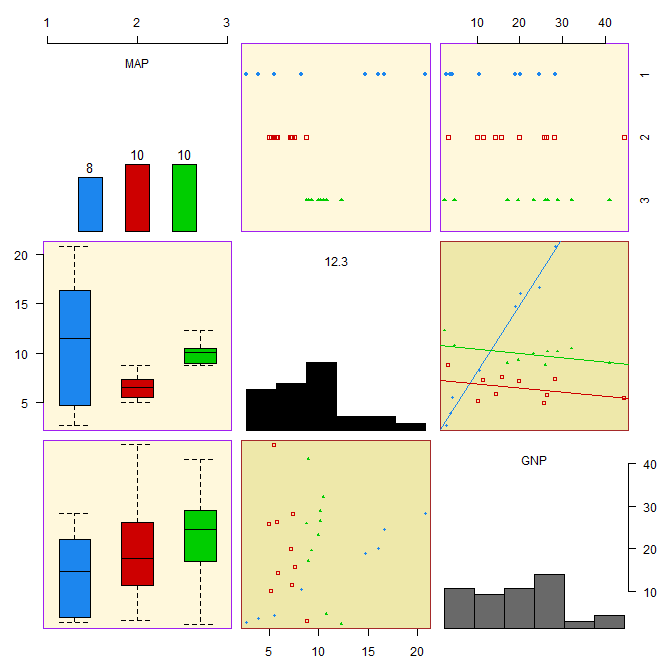Visualise the density of the mixture distribution.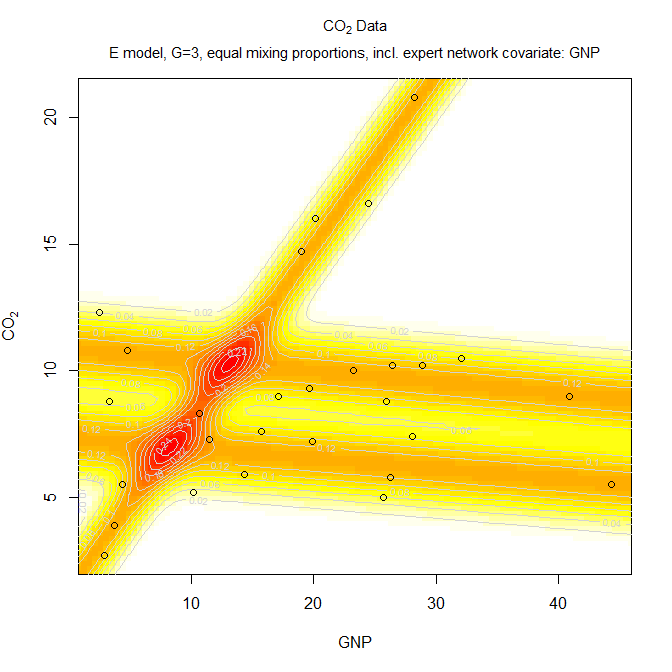Convert from the "MoEClust" class to the "Mclust" class in order to further visualise the results. Examine the "classification" and "uncertainty" options. mod <- as.Mclust(comp$optimal)
plot(mod, what="classification")
plot(mod, what="uncertainty")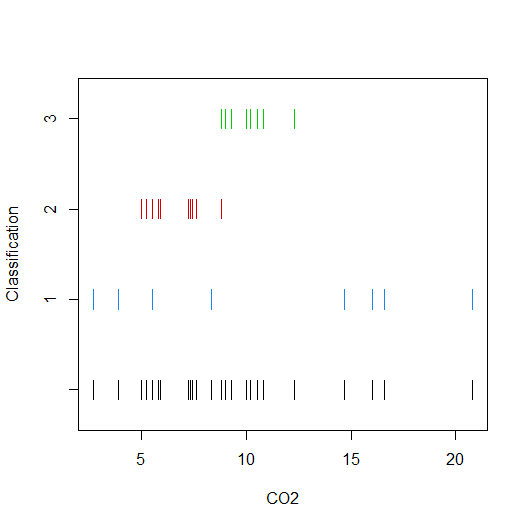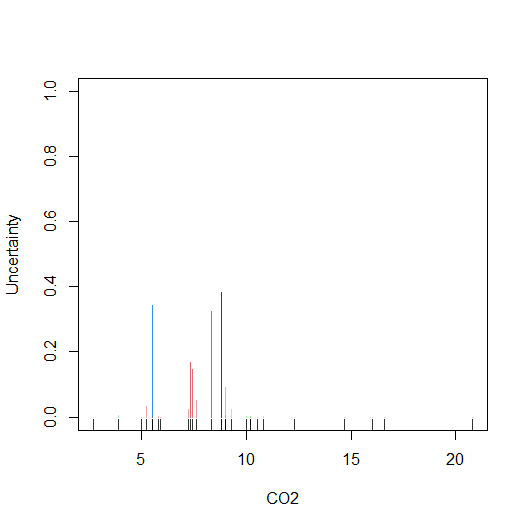Predictions can also be made from MoEClust models: the response, probability of cluster membership, and the MAP classification can be predicted for the fitted data or for new data (in the form of new covarariates and new response variables, or new covariates only). Let’s predict the response variable using the optimal model fit above to the CO2 data.

as.vector(predict(comp$optimal)$y)
##   14.258814  3.901403 20.461825  9.057476  8.292137 14.981877  6.849754  6.679807  9.695548 10.633012  9.451612  9.831250  6.255060  9.590722  7.237613  5.289370  9.782903  6.588625  9.531197 17.968482  8.514087  6.936512  6.725345  6.275746  5.546735  3.319411  9.911038 10.737124

Now let’s build a model on some of the CO2 data and retain the indices of the withheld observations:

ind     <- sample(1:nrow(CO2data), 2)
res2    <- MoE_clust(CO2data[-ind,]$CO2, G=3, expert=~GNP, equalPro=TRUE, network.data=CO2data[-ind,]) Now we can make predictions on the withheld data, either by using the withheld covariates only, or by also using the withheld response variables. Note that newdata can be either a list with component(s) new.x (and optionally new.y) or a single matrix/data.frame with the appropriate columns. predict(res2, newdata= list(new.x=CO2data[ind,"GNP", drop=FALSE])) # Using new covariates only ##$y
##         CO2
## 1 12.142620
## 2  9.399426
##
## $classification ## 1 2 ## 1 3 ## ##$z
##    Cluster1  Cluster2  Cluster3
## 1 0.3333333 0.3333333 0.3333333
## 2 0.3333333 0.3333333 0.3333333

predict(res2, newdata = CO2data[ind,])         # Using both new covariates & new response data
## $y ## CO2 ## 1 8.209032 ## 2 7.666103 ## ##$classification
## 1 2
## 2 3
##
## $z ## Cluster1 Cluster2 Cluster3 ## 1 2.609252e-57 0.9999765 0.0000235214 ## 2 8.124206e-07 0.2986029 0.7013963214 ## AIS Data Load the Australian Institute of Sports data. data(ais) hema <- ais[,3:7] Examine the various additional options around initialisation of the algorithm: ?MoE_control Fit a parsimonious Gaussian mixture of experts MoEClust model to the hematological variables within the AIS data, supplying sex in the expert network and BMI in the gating network via formula interfaces. Include an additional noise component by specifiying it’s prior mixing proportion tau0. Toggle between allowing the mixing proportion for the noise component depend on the gating concomitant or not via the noise.gate argument. This time, allow the printing of messages to the screen. mod <- MoE_clust(hema, G=1:3, expert= ~ sex, gating= ~ BMI, network.data=ais, tau0=0.1, noise.gate=FALSE) Visualise the results for the optimal model using a generalised pairs plot. plot(mod, what="gpairs")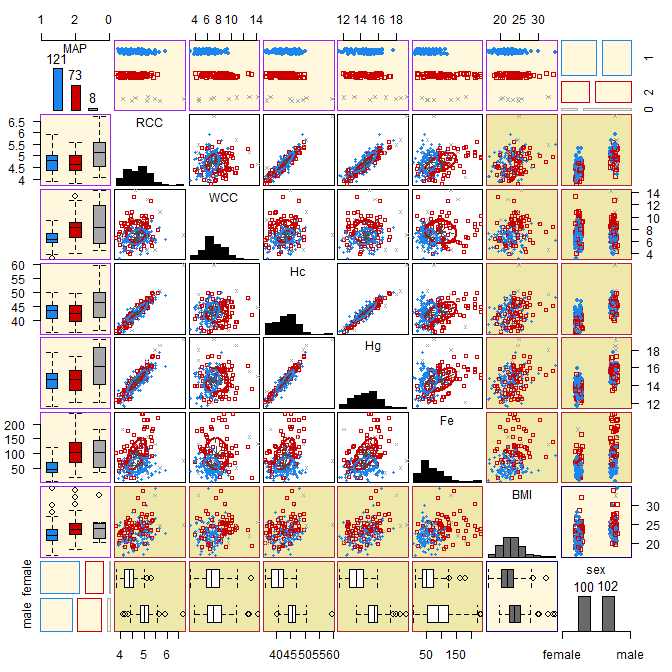Replace the scatter plots in response vs. response panels with bivariate density contours. Note that this is liable to be slow for models with expert network covariates. plot(mod, what="gpairs", response.type="density")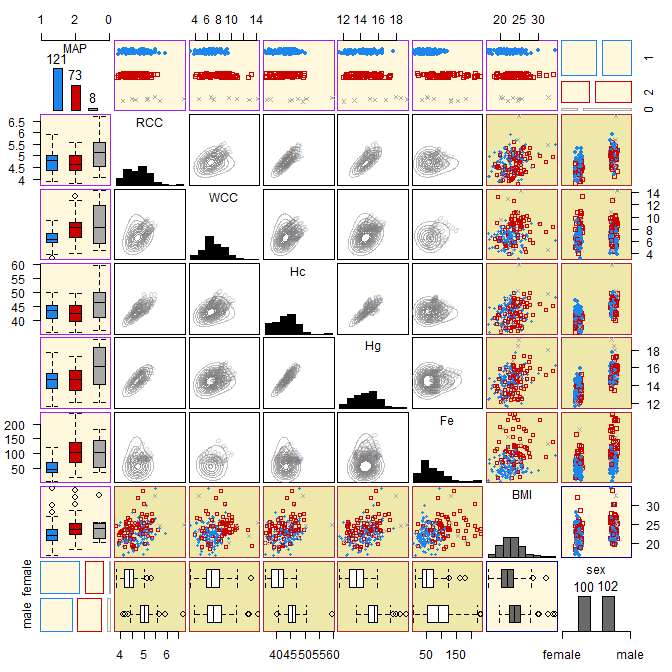Visualise the clustering uncertainty for the optimal model using a generalised pairs plot. plot(mod, what="gpairs", response.type="uncertainty")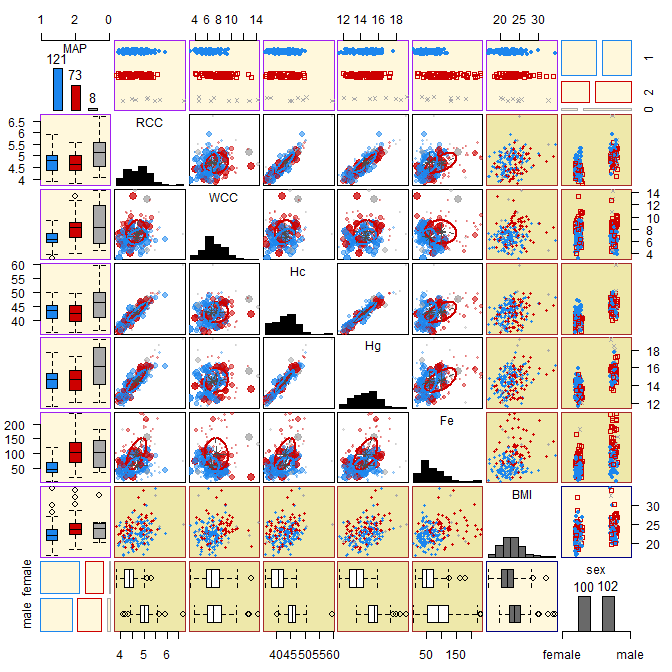Instead visualise the clustering uncertainty in the form of an ordered profile plot (type="barplot" can also be specified here). plot(mod, what="uncertainty", type="profile")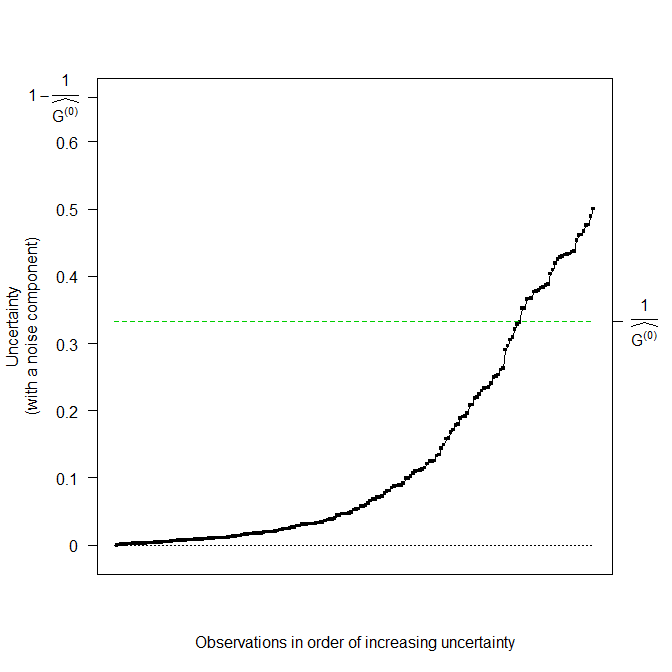Plot the BIC of the visited models. plot(mod, what="criterion")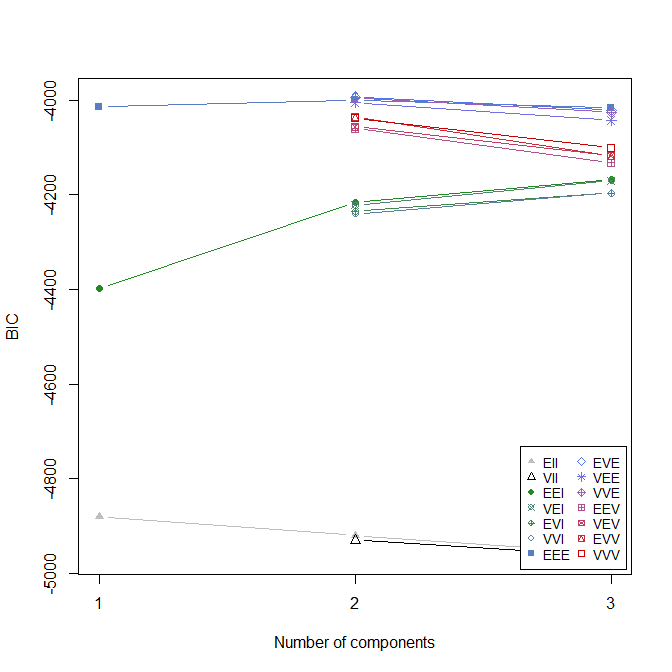Plot the gating network of the optimal model against the gating concomitant BMI. Note the horizontal line of grey points corresponding to the noise component due to the specification of noise.gate=FALSE in the original function call. plot(mod, what="gating", x.axis=ais$BMI, type="p", xlab="BMI", pch=1)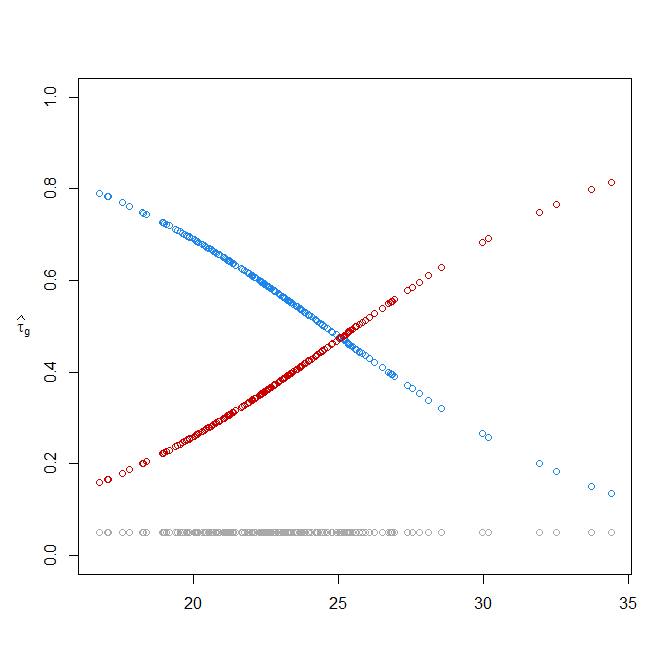For the optimal model, plot the log-likelihood vs. the number of EM iterations.

plot(mod, what="loglik")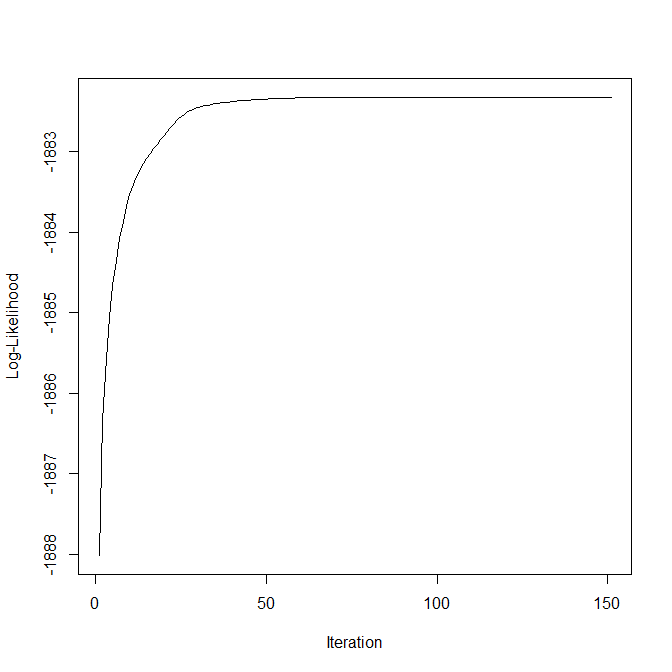Produce further visualisations for the Gaussian components with the aid of the lattice library.

require("lattice")
z <- factor(mod$classification[mod$classification > 0], labels=paste0("Cluster", seq_len(mod$G))) splom(~ hema | ais$sex, groups=z)
splom(~ hema | z, groups=ais\$sex)